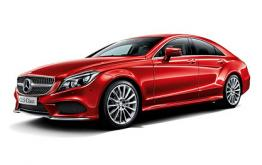# 奔驰(进口)奔驰CLS级

## 路跑女神测试 2015款奔驰CLS 400 4MATIC

2015年11月13日 11:10来源：www.chextx.com超过：17007次关注阅读全文

我自认为是一个取向正常、心理健康的男人，所以看到美女定会侧目斜视，然后继续保持镇定，顾左右而言他；那如果可以和自己喜欢的汽车来个亲密接触的话，我一定不会放过这个机会，必须“深入体验”一番。

我把奔驰CLS当做车中的女神，不知道你们是否同意我的观点。优雅曼妙的身材线条，雍容华贵，是我喜欢的类型，但我并不会爱上它，因为要俘获它的芳心代价并不一般，以车系中实力最强的CLS 400 4MATIC为例，最基本的版本就要98.8万元，而且还有各种选装配置。内容不少，我们还是先从外观说起。

新款CLS在前脸的造型上都一样，包括低配的CLS 260、320都拥有AMG风格外观套件，包括前脸夸张的进气口以及钻石型的中网。而这款CLS 400 4MATIC上还单独拥有AMG运动组件，比如19英寸AMG轮圈、直接控制(DIRECTCONTROL)运动型悬架以及平底多功能方向盘。

CLS 400 4MATIC标配了奔驰最新的多光束智能全LED大灯，在AUTO模式下它可以自动识别行驶的路况，从而选择最理想的灯光模式，例如碰到迎面来车或前方有车辆时，大灯可以在对方车辆上形成一个U型暗光模式，不会晃到对方驾驶员。我们从视频上看到它工作时的样子，就好像人类的眼睛。

车身尺寸方面CLS 400 4MATIC的长宽高分别为4956*1881*1423mm，轴距为2874mm，在同级别中属于最秀气的，除高度之外其他各项数值都不及宝马640i xDrive Gran Coupe以及奥迪A7 2014款 50 TFSI quattro 豪华型。

有着犀利的前脸，那么注定CLS的尾部不会低调，菱形的LED尾灯在夜晚点亮后更加妖艳妩媚，像是美女上妆后的眼线，容易让人产生向往。并且刹车灯光照强度在等红灯的时候会自动降低，避免对后方驾驶员造成眩目。这是一项非常人性化的设计，我想在奥迪车后面等过红灯的朋友都应该深有体会，无法直视！

CLS 400 4MATIC的轮胎为倍耐力的顶级运动型号P ZERO，尺寸达到了夸张的前255/35 R19，后285/30 R19，配合多辐AMG轮圈，视觉效果非常不错。说句题外话，我惊讶的发现只有211马力的CLS 260竟然也采用285mm宽度的后胎，动力感受真是让人捏把汗。

车身做工值得称赞，车外各个接缝均匀，需要用楔形塞尺才能发现发动机舱盖左右有1mm的差别，符合百万元豪华品牌的标准。

可以说CLS 400 4MATIC是那种能让人一见钟情的车型，善于吸引周围所有人的目光，偶然的接触就能让人印象深刻，使人不自觉地向往和喜欢，就算没有在第一时间打动你，“她”也可以让你日久生情，这也许就是大众情人的魅力。

#### 相关文章

0-500 字已有评论 0条 查看评论>>

### 奔驰CLS级### 猜你喜欢

﻿
• 快速找车
• 选择品牌
• 选择品牌
• A  奥迪
• A  阿斯顿·马丁
• A  阿尔法·罗密欧
• B  宝沃
• B  布加迪
• B  巴博斯
• B  保时捷
• B  宾利
• B  奔驰
• B  宝马
• B  本田
• B  别克
• B  标致
• B  比亚迪
• B  宝骏
• B  北汽制造
• B  北汽新能源
• B  北汽幻速
• B  北汽威旺
• B  北京汽车
• B  奔腾
• B  北汽绅宝
• C  长安
• C  长安商用
• C  长城
• C  昌河
• D  大众
• D  道奇
• D  DS
• D  东南
• D  东风风神
• D  东风风行
• D  东风小康
• D  东风风度
• D  东风
• F  福特
• F  丰田
• F  菲亚特
• F  法拉利
• F  福田
• F  福迪
• F  福汽启腾
• G  观致
• G  广汽传祺
• G  广汽吉奥
• G  GMC
• H  红旗
• H  汉腾汽车
• H  哈弗
• H  哈飞
• H  海格
• H  海马
• H  华颂
• H  黄海
• H  华泰
• H  恒天
• J  吉利汽车
• J  捷豹
• J  Jeep
• J  江淮
• J  江铃
• J  金杯
• J  九龙
• J  金旅
• K  凯翼
• K  凯迪拉克
• K  克莱斯勒
• K  科尼塞克
• K  卡威
• K  开瑞
• L  路虎
• L  林肯
• L  劳斯莱斯
• L  兰博基尼
• L  雷克萨斯
• L  铃木
• L  雷诺
• L  理念
• L  力帆
• L  莲花汽车
• L  猎豹
• L  路特斯
• L  陆风
• M  马自达
• M  MG
• M  MINI
• M  玛莎拉蒂
• M  摩根
• M  迈凯轮
• N  纳智捷
• O  欧宝
• O  讴歌
• O  欧朗
• Q  奇瑞
• Q  起亚
• Q  启辰
• R  日产
• R  荣威
• R  瑞麒
• S  三菱
• S  斯威汽车
• S  萨博
• S  smart
• S  斯柯达
• S  斯巴鲁
• S  思铭
• S  双龙
• S  上汽大通
• S  双环
• T  特斯拉
• T  腾势
• W  沃尔沃
• W  五菱汽车
• W  五十铃
• W  威兹曼
• W  威麟
• X  现代
• X  雪佛兰
• X  雪铁龙
• X  西雅特
• Y  一汽
• Y  英菲尼迪
• Y  英致
• Y  依维柯
• Y  野马汽车
• Y  永源
• Z  众泰
• Z  中华
• Z  中兴
• Z  知豆
• 选择车系
• 选择车系
• 车型对比
• 选择品牌
• 选择品牌
• A  奥迪
• A  阿斯顿·马丁
• A  阿尔法·罗密欧
• B  宝沃
• B  布加迪
• B  巴博斯
• B  保时捷
• B  宾利
• B  奔驰
• B  宝马
• B  本田
• B  别克
• B  标致
• B  比亚迪
• B  宝骏
• B  北汽制造
• B  北汽新能源
• B  北汽幻速
• B  北汽威旺
• B  北京汽车
• B  奔腾
• B  北汽绅宝
• C  长安
• C  长安商用
• C  长城
• C  昌河
• D  大众
• D  道奇
• D  DS
• D  东南
• D  东风风神
• D  东风风行
• D  东风小康
• D  东风风度
• D  东风
• F  福特
• F  丰田
• F  菲亚特
• F  法拉利
• F  福田
• F  福迪
• F  福汽启腾
• G  观致
• G  广汽传祺
• G  广汽吉奥
• G  GMC
• H  红旗
• H  汉腾汽车
• H  哈弗
• H  哈飞
• H  海格
• H  海马
• H  华颂
• H  黄海
• H  华泰
• H  恒天
• J  吉利汽车
• J  捷豹
• J  Jeep
• J  江淮
• J  江铃
• J  金杯
• J  九龙
• J  金旅
• K  凯翼
• K  凯迪拉克
• K  克莱斯勒
• K  科尼塞克
• K  卡威
• K  开瑞
• L  路虎
• L  林肯
• L  劳斯莱斯
• L  兰博基尼
• L  雷克萨斯
• L  铃木
• L  雷诺
• L  理念
• L  力帆
• L  莲花汽车
• L  猎豹
• L  路特斯
• L  陆风
• M  马自达
• M  MG
• M  MINI
• M  玛莎拉蒂
• M  摩根
• M  迈凯轮
• N  纳智捷
• O  欧宝
• O  讴歌
• O  欧朗
• Q  奇瑞
• Q  起亚
• Q  启辰
• R  日产
• R  荣威
• R  瑞麒
• S  三菱
• S  斯威汽车
• S  萨博
• S  smart
• S  斯柯达
• S  斯巴鲁
• S  思铭
• S  双龙
• S  上汽大通
• S  双环
• T  特斯拉
• T  腾势
• W  沃尔沃
• W  五菱汽车
• W  五十铃
• W  威兹曼
• W  威麟
• X  现代
• X  雪佛兰
• X  雪铁龙
• X  西雅特
• Y  一汽
• Y  英菲尼迪
• Y  英致
• Y  依维柯
• Y  野马汽车
• Y  永源
• Z  众泰
• Z  中华
• Z  中兴
• Z  知豆
• 选择车系
• 选择车系
• 选择车型
• 选择车型
• 意见反馈